﻿ 一类具有双时滞效应的氮–磷–浮游植物模型的动力学研究 Dynamic Analysis of a Nitrogen-Phosphorus-Plankton Model with Two Time Delays

Vol.07 No.08(2018), Article ID:26660,14 pages
10.12677/AAM.2018.78128

Dynamic Analysis of a Nitrogen-Phosphorus-Plankton Model with Two Time Delays

Pan Zhao

Wenzhou University, Wenzhou Zhejiang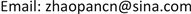Received: Aug. 10th, 2018; accepted: Aug. 23rd, 2018; published: Aug. 30th, 2018ABSTRACT

Under the framework of water eutrophication prevention and control research, a nitrogen-phosphorus-phytoplankton model with two delays will be investigated mathematically and numerically. Mathematical works have comprehensively explored the locally asymptotic stability of positive equilibrium and the threshold conditions of occurring Hopf bifurcation. Numerical simulation works have depicted the dynamic evolution process of nutrient and phytoplankton density and Hopf bifurcation. These results provide a great help for the interaction between nutrition and phytoplankton in dynamics, and are helpful to deeply understand how the delay affects the dynamic trend of the marine ecosystem. Furthermore, they can provide certain theoretical support for further prevention and control of eutrophication of water bodies as well.

Keywords:Nutrient, Phytoplankton, Delay, Stability, Hopf Bifurcation1. 引言

Li and Liu  研究了一类具有时滞效应的浮游生物模型，解析出系统正平衡点全局稳定性和发生Hopf分岔的阈值条件，这一方法很值得我们借鉴。Martin and Ruan  考虑了收获率和时间延迟的综合影响，构建了一类带有时滞效应和选择性收获的浮游植物–浮游动物模型，他们发现时滞因素可能会导致系统发生失稳，但是当收获值处于临界收获水平时，收获率对系统的平衡有稳定作用，这项工作可以为研究收获率和时间延迟之间的动态机制提供一个良好的框架。Shi et al.  研究了一类具有分布时滞和Dirichlet边界条件的反应–扩散模型，他们的研究成果具有非常重要的意义，为我们研究营养循环如何影响浮游植物生长的问题提供了一套系统的研究方法。Shi and Jiang  研究了一类具有双时滞效应的浮游动物–浮游植物模型，这一研究为我们以后研究多时滞模型提供了一套完整的理论和模拟方法等。他们的研究成果对预防和控制水体的富营养化具有一定的指导意义。

$\left\{\begin{array}{l}\frac{\text{d}x}{\text{d}t}={I}_{1}-{b}_{1}x-\frac{{a}_{1}xz}{{c}_{1}+x}\\ \frac{\text{d}y}{\text{d}t}={I}_{2}-{b}_{2}y-\frac{{a}_{2}yz}{{c}_{2}+y}\\ \frac{\text{d}z}{\text{d}t}=\frac{{e}_{1}{a}_{1}x\left(t-{\tau }_{1}\right)z}{{c}_{1}+x\left(t-{\tau }_{1}\right)}+\frac{{e}_{2}{a}_{2}y\left(t-{\tau }_{2}\right)z}{{c}_{2}+y\left(t-{\tau }_{2}\right)}-mz\end{array}$ , (1)

$\begin{array}{l}x\left(t\right)={\varphi }_{1}\left(\theta \right)\ge 0\\ y\left(t\right)={\varphi }_{2}\left(\theta \right)\ge 0\\ z\left(t\right)={\varphi }_{3}\left(\theta \right)\ge 0\end{array}\right\}\text{\hspace{0.17em}}\text{\hspace{0.17em}}\text{\hspace{0.17em}}\theta \in \left[-\tau ,0\right]$ , (2)

2. 局部稳定性和Hopf分支

$\frac{\text{d}U}{\text{d}t}=MU\left(t\right)+NU\left(t-{\tau }_{1}\right)+OU\left(t-{\tau }_{2}\right)$ , (3)

$U\left(t\right)={\left[u,v,w\right]}^{\text{T}}$ , $M={\left({m}_{ij}\right)}_{3×3}$ , $N={\left({n}_{ij}\right)}_{3×3}$ , $O={\left({o}_{ij}\right)}_{3×3}$ ,

${m}_{11}=-{b}_{1}-\frac{{a}_{1}{c}_{1}{z}^{*}}{{\left({c}_{1}+{x}^{*}\right)}^{2}}$ , ${m}_{13}=-\frac{{a}_{1}{x}^{*}}{{c}_{1}+{x}^{*}}$ ,

${m}_{22}=-{b}_{2}-\frac{{a}_{2}{c}_{2}{z}^{*}}{{\left({c}_{2}+{y}^{*}\right)}^{2}}$ , ${m}_{23}=-\frac{{a}_{2}{y}^{*}}{{c}_{2}+{y}^{*}}$ ,

${n}_{31}=\frac{{e}_{1}{a}_{1}{c}_{1}{z}^{*}}{{\left({c}_{1}+{x}^{*}\right)}^{2}}$ , ${n}_{32}=\frac{{e}_{2}{a}_{2}{c}_{2}{z}^{*}}{{\left({c}_{2}+{y}^{*}\right)}^{2}}$ ,

$|\begin{array}{ccc}\lambda -{a}_{11}& 0& -{a}_{13}\\ 0& \lambda -{a}_{22}& -{a}_{23}\\ -{a}_{31}\cdot {\text{e}}^{-\lambda {\tau }_{1}}& -{a}_{32}\cdot {\text{e}}^{-\lambda {\tau }_{2}}& \lambda \end{array}|=0$ , (4)

${a}_{11}=-{b}_{1}-\frac{{a}_{1}{c}_{1}{z}^{*}}{{\left({c}_{1}+{x}^{*}\right)}^{2}}$ , ${a}_{13}=-\frac{{a}_{1}{x}^{*}}{{c}_{1}+{x}^{*}}$ ,

${a}_{22}=-{b}_{2}-\frac{{a}_{2}{c}_{2}{z}^{*}}{{\left({c}_{2}+{y}^{*}\right)}^{2}}$ , ${a}_{23}=-\frac{{a}_{2}{y}^{*}}{{c}_{2}+{y}^{*}}$ ,

${a}_{31}=\frac{{e}_{1}{a}_{1}{c}_{1}{z}^{*}}{{\left({c}_{1}+{x}^{*}\right)}^{2}}$ , ${a}_{32}=\frac{{e}_{2}{a}_{2}{c}_{2}{z}^{*}}{{\left({c}_{2}+{y}^{*}\right)}^{2}}$ ,

${\lambda }^{3}+A{\lambda }^{2}+B\lambda +\left(C+D\lambda \right){\text{e}}^{-\lambda {\tau }_{1}}+\left(E+F\lambda \right){\text{e}}^{-\lambda {\tau }_{2}}=0$ , (5)

$A=-\left({a}_{11}+{a}_{22}\right)$ , $B={a}_{11}{a}_{22}$ , $B={a}_{11}{a}_{22}$ ,

$D=-{a}_{13}{a}_{31}$ , $D=-{a}_{13}{a}_{31}$ , $D=-{a}_{13}{a}_{31}$ .

${\lambda }^{3}+{A}_{11}{\lambda }^{2}+{A}_{12}\lambda +{A}_{13}=0$ , (6)

${A}_{11}=A$ , ${A}_{12}=B+D+F$ , ${A}_{13}=C+E$ .

1) $\left({H}_{21}\right)$ ：文献  中定理(2.1)情况(i)，(iii)成立；

2) $\left({H}_{22}\right)$${{f}^{\prime }}_{1}\left({\omega }_{10}^{2}\right)\ne 0$${A}_{21}^{2}-2{A}_{22}>0$${A}_{22}^{2}-2{A}_{21}{A}_{23}>0$

${\lambda }^{3}+{A}_{21}{\lambda }^{2}+{A}_{22}\lambda +{A}_{23}+\left({B}_{21}\lambda +{B}_{22}\right){\text{e}}^{-\lambda {\tau }_{1}}=0$ , (7)

${A}_{21}=-\left({a}_{11}+{a}_{22}\right)$ , ${A}_{22}={a}_{11}{a}_{22}-{a}_{23}{a}_{32}$ , ${A}_{23}={a}_{23}{a}_{32}{a}_{11}$ ,

${B}_{21}=-{a}_{13}{a}_{31}$ , ${B}_{22}={a}_{13}{a}_{31}{a}_{22}$ ,

$\left\{\begin{array}{l}-{\omega }_{1}^{3}+{A}_{22}{\omega }_{1}+{B}_{21}{\omega }_{1}\mathrm{cos}\left({\omega }_{1}{\tau }_{1}\right)-{B}_{22}\mathrm{sin}\left({\omega }_{1}{\tau }_{1}\right)=0\\ -{A}_{21}{\omega }_{1}^{2}+{A}_{23}+{B}_{21}{\omega }_{1}\mathrm{sin}\left({\omega }_{1}{\tau }_{1}\right)+{B}_{22}\mathrm{cos}\left({\omega }_{1}{\tau }_{1}\right)=0\end{array}$ , (8)

${\omega }_{1}^{6}+{E}_{21}{\omega }_{1}^{4}+{E}_{22}{\omega }_{1}^{2}+{E}_{23}=0$ ,(9)

${E}_{21}={A}_{21}^{2}-2{A}_{22}$ , ${E}_{22}={A}_{22}^{2}-{B}_{21}^{2}-2{A}_{21}{A}_{23}$ , ${E}_{23}={A}_{23}^{2}-{B}_{22}^{2}$ .

${v}_{1}^{3}+{E}_{21}{v}_{1}^{2}+{E}_{22}{v}_{1}+{E}_{23}=0$ . (10)

${f}_{1}\left({v}_{1}\right)={v}_{1}^{3}+{E}_{21}{v}_{1}^{2}+{E}_{22}{v}_{1}+{E}_{23}$ . (11)

$\mathrm{cos}\left({\omega }_{1}{\tau }_{1}\right)=\frac{{B}_{21}{\omega }_{1}^{4}+\left({B}_{22}{A}_{21}-{B}_{21}{A}_{22}\right){\omega }_{1}^{2}-{B}_{22}{A}_{23}}{{B}_{21}^{2}{\omega }_{1}^{2}+{B}_{22}^{2}}$ , (12)

$\mathrm{sin}\left({\omega }_{1}{\tau }_{1}\right)=\frac{\left({B}_{21}{A}_{21}-{B}_{22}\right){\omega }_{1}^{3}+\left({B}_{22}{A}_{22}-{B}_{21}{A}_{23}\right){\omega }_{1}}{{B}_{21}^{2}{\omega }_{1}^{2}+{B}_{22}^{2}}$ , (13)

${\phi }_{1}=\left\{\begin{array}{l}2\text{π}-\mathrm{arccos}\left[\frac{{B}_{21}{\omega }_{1}^{4}+\left({B}_{22}{A}_{21}-{B}_{21}{A}_{22}\right){\omega }_{1}^{2}-{B}_{22}{A}_{23}}{{B}_{21}^{2}{\omega }_{1}^{2}+{B}_{22}^{2}}\right],\text{\hspace{0.17em}}\text{\hspace{0.17em}}\text{if}\text{\hspace{0.17em}}\left(13\right)<0\\ \mathrm{cos}\left[\frac{{B}_{21}{\omega }_{1}^{4}+\left({B}_{22}{A}_{21}-{B}_{21}{A}_{22}\right){\omega }_{1}^{2}-{B}_{22}{A}_{23}}{{B}_{21}^{2}{\omega }_{1}^{2}+{B}_{22}^{2}}\right],\text{\hspace{0.17em}}\text{\hspace{0.17em}}\text{if}\text{\hspace{0.17em}}\left(13\right)\ge 0\end{array}$ , (14)

${\tau }_{1k}^{j}=\frac{1}{{\omega }_{1k}}\left({\phi }_{1}+2j\text{π}\right),\left(j=0,1,2,\cdots ,k=1,2,3\right)$ ,(15)

${\left(\frac{\text{d}\lambda }{\text{d}{\tau }_{1}}\right)}^{-1}=\frac{3{\lambda }^{2}+2{A}_{21}\lambda +{A}_{22}}{-\lambda \left({\lambda }^{3}+{A}_{21}{\lambda }^{2}+{A}_{22}\lambda +{A}_{23}\right)}+\frac{{B}_{21}}{{B}_{21}{\lambda }^{2}+{B}_{22}\lambda }-\frac{{\tau }_{1}}{\lambda }$ , (16)

$\begin{array}{c}{\left(\frac{\text{d}\mathrm{Re}\left(\lambda \right)}{\text{d}{\tau }_{1}}\right)}_{\lambda =i{\omega }_{10}}^{-1}=\frac{3{\omega }_{10}^{6}+2\left({A}_{21}^{2}-2{A}_{22}\right){\omega }_{10}^{4}+\left({A}_{22}^{2}-{B}_{21}^{2}-2{A}_{21}{A}_{23}\right){\omega }_{10}^{2}}{{\omega }_{10}^{8}+\left({A}_{21}^{2}-2{A}_{22}\right){\omega }_{10}^{6}+\left({A}_{22}^{2}-2{A}_{21}{A}_{23}\right){\omega }_{10}^{4}+{A}_{23}^{2}{\omega }_{10}^{2}}\\ =\frac{1}{{\omega }_{10}^{2}}\left(\frac{{{f}^{\prime }}_{1}\left({\omega }_{10}^{2}\right)}{{\omega }_{10}^{6}+\left({A}_{21}^{2}-2{A}_{22}\right){\omega }_{10}^{4}+\left({A}_{22}^{2}-2{A}_{21}{A}_{23}\right){\omega }_{10}^{2}+{A}_{23}^{2}}\right),\end{array}$ (17)

1) $\left({H}_{21}\right)$ ：同定理2.2；

2) $\left({H}_{32}\right)$${{f}^{\prime }}_{2}\left({\omega }_{20}^{2}\right)\ne 0$${A}_{31}^{2}-2{A}_{32}>0$${A}_{32}^{2}-2{A}_{31}{A}_{33}>0$

${\lambda }^{3}+{A}_{31}{\lambda }^{2}+{A}_{32}\lambda +{A}_{33}+\left({B}_{31}\lambda +{B}_{32}\right){\text{e}}^{-\lambda {\tau }_{2}}=0$ , (18)

${A}_{31}=-\left({a}_{11}+{a}_{22}\right)$ , ${A}_{32}={a}_{11}{a}_{22}-{a}_{13}{a}_{31}$ , ${A}_{33}={a}_{13}{a}_{31}{a}_{22}$ , ${B}_{31}=-{a}_{23}{a}_{32}$ , ${B}_{32}={a}_{23}{a}_{32}{a}_{11}$ ,

$\left\{\begin{array}{c}-{\omega }_{2}^{3}+{A}_{32}{\omega }_{2}+{B}_{31}{\omega }_{2}\mathrm{cos}\left({\omega }_{2}{\tau }_{2}\right)-{B}_{32}\mathrm{sin}\left({\omega }_{2}{\tau }_{2}\right)=0\\ -{A}_{31}{\omega }_{2}^{2}+{A}_{33}+{B}_{31}{\omega }_{2}\mathrm{sin}\left({\omega }_{2}{\tau }_{2}\right)+{B}_{32}\mathrm{cos}\left({\omega }_{2}{\tau }_{2}\right)=0\end{array}$ , (19)

${\omega }_{2}^{6}+{E}_{31}{\omega }_{2}^{4}+{E}_{32}{\omega }_{2}^{2}+{E}_{33}=0$ , (20)

${E}_{31}={A}_{31}^{2}-2{A}_{32}$ , ${E}_{32}={A}_{32}^{2}-{B}_{31}^{2}-2{A}_{31}{A}_{33}$ , ${E}_{33}={A}_{33}^{2}-{B}_{32}^{2}$ .

${v}_{2}^{3}+{E}_{31}{v}_{2}^{2}+{E}_{32}{v}_{2}+{E}_{33}=0$ . (21)

${f}_{2}\left({v}_{2}\right)={v}_{2}^{3}+{E}_{31}{v}_{2}^{2}+{E}_{32}{v}_{2}+{E}_{33}$ . (22)

$\mathrm{cos}\left({\omega }_{2}{\tau }_{2}\right)=\frac{{B}_{31}{\omega }_{2}^{4}+\left({B}_{32}{A}_{31}-{B}_{31}{A}_{32}\right){\omega }_{2}^{2}-{B}_{32}{A}_{33}}{{B}_{31}^{2}{\omega }_{2}^{2}+{B}_{32}^{2}}$ , (23)

$\mathrm{sin}\left({\omega }_{2}{\tau }_{2}\right)=\frac{\left({B}_{31}{A}_{31}-{B}_{32}\right){\omega }_{2}^{3}+\left({B}_{32}{A}_{32}-{B}_{31}{A}_{33}\right){\omega }_{2}}{{B}_{31}^{2}{\omega }_{2}^{2}+{B}_{32}^{2}}$ , (24)

${\phi }_{2}=\left\{\begin{array}{l}2\text{π}-\mathrm{arccos}\left[\frac{{B}_{31}{\omega }_{2}^{4}+\left({B}_{32}{A}_{31}-{B}_{31}{A}_{32}\right){\omega }_{2}^{2}-{B}_{32}{A}_{33}}{{B}_{31}^{2}{\omega }_{2}^{2}+{B}_{32}^{2}}\right],\text{\hspace{0.17em}}\text{\hspace{0.17em}}\text{if}\text{\hspace{0.17em}}\left(24\right)<0\\ \mathrm{cos}\left[\frac{{B}_{31}{\omega }_{2}^{4}+\left({B}_{32}{A}_{31}-{B}_{31}{A}_{32}\right){\omega }_{2}^{2}-{B}_{32}{A}_{33}}{{B}_{31}^{2}{\omega }_{2}^{2}+{B}_{32}^{2}}\right],\text{\hspace{0.17em}}\text{\hspace{0.17em}}\text{if}\text{\hspace{0.17em}}\left(24\right)\ge 0\end{array}$ ,

${\tau }_{2k}^{j}=\frac{1}{{\omega }_{2k}}\left({\phi }_{2}+2j\text{π}\right),\left(j=0,1,2,\cdots ,k=1,2,3\right)$ . (25)

${\left(\frac{\text{d}\lambda }{\text{d}{\tau }_{2}}\right)}^{-1}=\frac{3{\lambda }^{2}+2{A}_{31}\lambda +{A}_{32}}{-\lambda \left({\lambda }^{3}+{A}_{31}{\lambda }^{2}+{A}_{32}\lambda +{A}_{33}\right)}+\frac{{B}_{31}}{{B}_{31}{\lambda }^{2}+{B}_{32}\lambda }-\frac{{\tau }_{2}}{\lambda }$ , (26)

${\left(\frac{\text{d}\mathrm{Re}\left(\lambda \right)}{\text{d}{\tau }_{2}}\right)}_{\lambda =i{\omega }_{20}}^{-1}=\frac{1}{{\omega }_{20}^{2}}\left(\frac{{{f}^{\prime }}_{2}\left({\omega }_{20}^{2}\right)}{{\omega }_{20}^{6}+\left({A}_{31}^{2}-2{A}_{32}\right){\omega }_{20}^{4}+\left({A}_{32}^{2}-2{A}_{31}{A}_{33}\right){\omega }_{20}^{2}+{A}_{33}^{2}}\right)$ , (27)

1) $\left({H}_{41}\right)$ ：(29)存在有限个正根；

2) $\left({H}_{42}\right)$$\left({A}_{1}{C}_{1}+{B}_{1}{D}_{1}\right)\left({\omega }_{20}^{\ast }{}^{2}+{a}_{11}^{2}\right)-\left({A}_{1}^{2}+{B}_{1}^{2}\right)\ne 0$

$\left\{\begin{array}{l}-{\omega }_{2}^{\ast }{}^{3}+{a}_{11}{a}_{22}{\omega }_{2}^{\ast }-\left[{a}_{13}{a}_{31}{a}_{22}\mathrm{sin}\left({\omega }_{2}^{\ast }{\tau }_{1}\right)+{a}_{13}{a}_{31}{\omega }_{2}^{\ast }\mathrm{cos}\left({\omega }_{2}^{\ast }{\tau }_{1}\right)\right]={a}_{23}{a}_{32}{a}_{11}\mathrm{sin}\left({\omega }_{2}^{\ast }{\tau }_{2}\right)+{a}_{23}{a}_{32}{\omega }_{2}^{\ast }\mathrm{cos}\left({\omega }_{2}^{\ast }{\tau }_{2}\right)\\ \left({a}_{11}+{a}_{22}\right){\omega }_{2}^{\ast }{}^{2}++{a}_{13}{a}_{31}{a}_{22}\mathrm{cos}\left({\omega }_{2}^{\ast }{\tau }_{1}\right)-{a}_{13}{a}_{31}{\omega }_{2}^{\ast }\mathrm{sin}\left({\omega }_{2}^{\ast }{\tau }_{1}\right)=-{a}_{23}{a}_{32}{a}_{11}\mathrm{cos}\left({\omega }_{2}^{\ast }{\tau }_{2}\right)+{a}_{23}{a}_{32}{\omega }_{2}^{\ast }\mathrm{sin}\left({\omega }_{2}^{\ast }{\tau }_{2}\right)\end{array}$ , (28)

${\omega }_{2}^{\ast }{}^{6}+{E}_{41}{\omega }_{2}^{\ast }{}^{4}+{E}_{42}{\omega }_{2}^{\ast }{}^{2}+{E}_{43}+{E}_{44}\mathrm{sin}\left({\omega }_{2}^{\ast }{\tau }_{1}\right)+{E}_{45}\mathrm{cos}\left({\omega }_{2}^{\ast }{\tau }_{1}\right)=0$ , (29)

${E}_{41}={a}_{11}^{2}+{a}_{22}^{2}$ , ${E}_{42}={a}_{13}^{2}{a}_{31}^{2}-{a}_{23}^{2}{a}_{32}^{2}+{a}_{11}^{2}{a}_{22}^{2}$ , ${E}_{43}={a}_{13}^{2}{a}_{31}^{2}{a}_{22}^{2}-{a}_{23}^{2}{a}_{32}^{2}{a}_{11}^{2}$ ,

${E}_{44}=-2{a}_{13}{a}_{31}{a}_{11}\left({\omega }_{2}^{\ast }{}^{3}+{a}_{22}^{2}{\omega }_{2}^{\ast }\right)$ , ${E}_{45}=2{a}_{13}{a}_{31}\left({\omega }_{2}^{\ast }{}^{4}+{a}_{22}^{2}{\omega }_{2}^{\ast }{}^{2}\right)$ .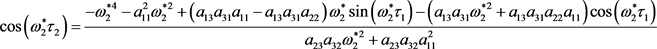,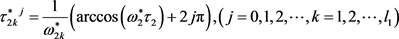, (30)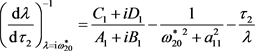,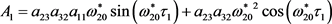,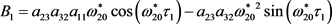,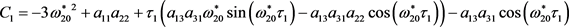,., (31)

1)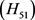：(33)存在有限个正根；

2)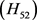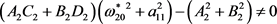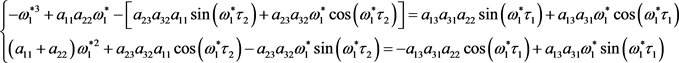, (32)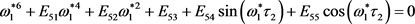, (33)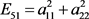,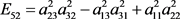,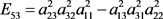,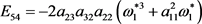,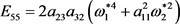.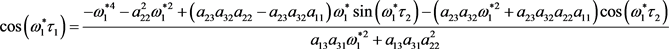,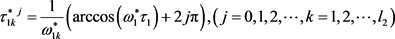, (34)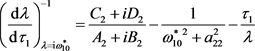,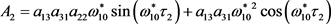,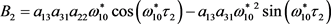,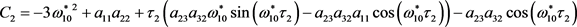,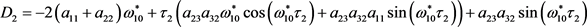., (35)

3. 数值仿真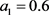,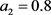,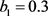,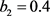,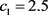,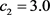,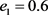,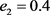,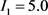,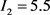,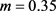.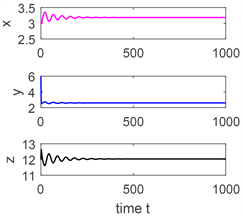(a)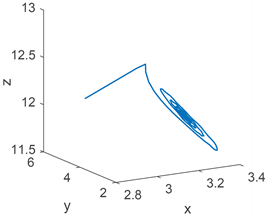(b)

Figure 1. (a) Time series; (b) Phase portrait, the initial value is (3, 3, 10), when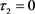,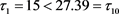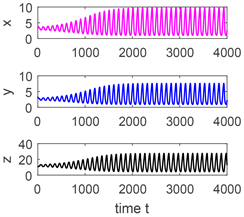(a)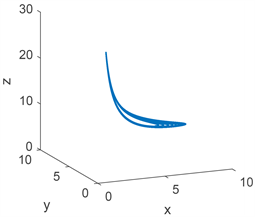(b)

Figure 2. (a) Time series; (b) Phase portrait, the initial value is (3, 3, 10), when,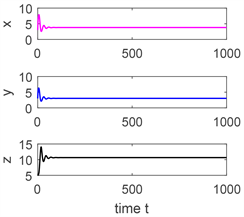(a)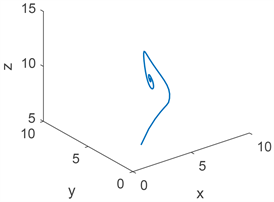(b)

Figure 3. (a) Time series; (b) Phase portrait, the initial value is (3, 3, 5), when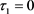,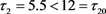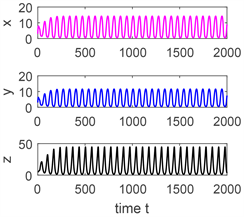(a)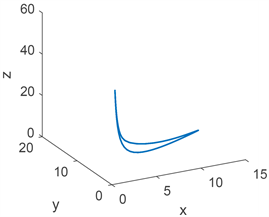(b)

Figure 4. (a) Time series; (b) Phase portrait, the initial value is (3, 3, 5), when,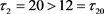(a)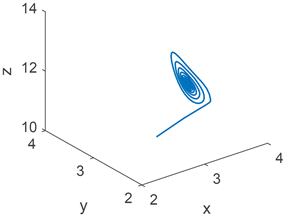(b)

Figure 5. (a) Time series; (b) Phase portrait, the initial value is (3, 3, 10), when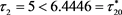,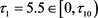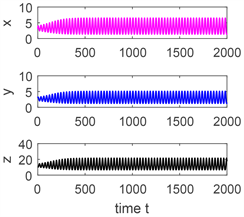(a)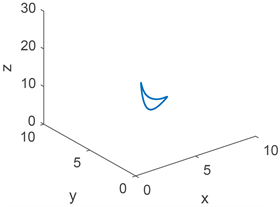(b)

Figure 6. (a) Time series; (b) Phase portrait, the initial value is (3, 3, 10), when,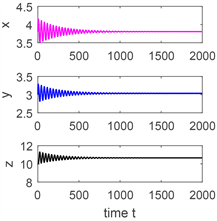(a)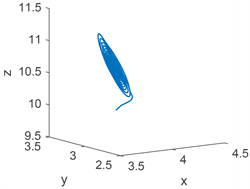(b)

Figure 7. (a) Time series; (b) Phase portrait, the initial value is (3, 3, 5), when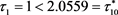,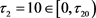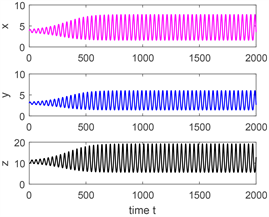(a)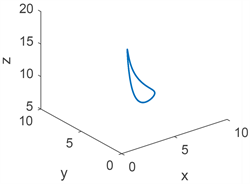(b)

Figure 8. (a) Time series; (b) Phase portrait, the initial value is (3, 3, 5), when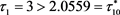,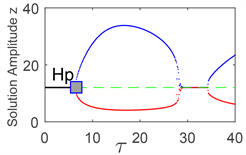(a)(b)

Figure 9. (a) Bifurcation diagram with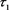and z, the initial value is (3, 3, 5), when; (b) Bifurcation diagram with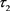and z, the initial value is (3, 3, 10), when4. 结论

Dynamic Analysis of a Nitrogen-Phosphorus-Plankton Model with Two Time Delays[J]. 应用数学进展, 2018, 07(08): 1105-1118. https://doi.org/10.12677/AAM.2018.78128

1. 1. Dai, C. Zhao, M. and Yu, H. (2016) Dynamics Induced by Delay in a Nutrient-Phytoplankton Model with Diffusion. Ecological Complexity, 26, 29-36. https://doi.org/10.1016/j.ecocom.2016.03.001

2. 2. Song, X. and Chen, L. (2001) Optimal Harvesting and Stability for a Two-Species Competitive System with Stage Structure. Mathematical Biosciences, 170, 173-186. https://doi.org/10.1016/S0025-5564(00)00068-7

3. 3. Ruan, S. and Wei, J. (2003) On the Zeros of Transcendental Functions with Applications to Stability of Delay Differential Equations with Two Delays. Dynamics of Continuous, Discrete and Impulsive Systems Series A: Mathematical Analysis, 10, 863-874.

4. 4. Laukkanen, M. and Huhtala, A. (2008) Optimal Management of a EUTROPHIED COASTAL ECOSYStem: Balancing agricultural and Municipal Abatement Measures. Environmental and Resource Economics, 39, 139-159. https://doi.org/10.1007/s10640-007-9099-2

5. 5. Ruan, S. (1995) The Effect of Delays on Stability and Persistence in Plankton Models. Nonlinear Analysis, 24, 575-585. https://doi.org/10.1016/0362-546X(95)93092-I

6. 6. Qin, B. (2009) Lake Eutrophication: Control Countermeasures and Recycling Exploitation. Ecological Engineering, 35, 1569-1573. https://doi.org/10.1016/j.ecoleng.2009.04.003

7. 7. Reigada, R., Hillary, R.M., Bees, M.A., Sancho, J.M. and Sagues, F. (2003) Plankton Blooms Induced by Turbulent Flows. Proceedings of the Royal Society B: Biological Sciences, 270, 875-880. https://doi.org/10.1098/rspb.2002.2298

8. 8. Gourley, S. A. and Ruan, S. (2003) Spatio-Temporal Delays in a Nutrient-Plankton Model on a Finite Domain: Linear Stability and Bifurcations. Applied Mathematics and Computation, 145, 391-412. https://doi.org/10.1016/S0096-3003(02)00494-0

9. 9. Zhao, M., Yu, H. and Zhu, J. (2009) Effects of a Population Floor on the Persistence of Chaos in a Mutual Interference Host-Parasitoid Model. Chaos, Solitons and Fractals, 42, 1245-1250. https://doi.org/10.1016/j.chaos.2009.03.027

10. 10. Yu, H., Zhao, M. and Agarwal, R.P. (2014) Stability and Dynamics Analysis of Time Delayed Eutrophication Ecological Model Based upon the Zeya Reservoir. Mathematics and Computers in Simulation, 97, 53-67. https://doi.org/10.1016/j.matcom.2013.06.008

11. 11. Mei, L. and Zhang, X. (2012) Existence and Nonexistence of Positive Steady States in Multi-Species Phytoplankton Dynamics. Journal of Differential Equations, 253, 2025-2063. https://doi.org/10.1016/j.jde.2012.06.011

12. 12. Wang, Y., Jiang, W. and Wang, H. (2013) Stability and Global Hopf Bifurcation in Toxic Phytoplankton-Zooplankton Model with Delay and Selective Harvesting. Nonlinear Dynamics, 73, 881-896. https://doi.org/10.1007/s11071-013-0839-2

13. 13. Wang, B., Zhao, M., Dai, C., Yu, H., Wang, N. and Wang, P. (2016) Dynamics Analysis of a Nutrient-Plankton Model with a Time Delay. Discrete Dynamics in Nature and Society, 2016, Article ID: 9797624. https://doi.org/10.1155/2016/9797624

14. 14. He, X. and Ruan, S. (1998) Global Stability in Chemostat-Type Plankton Models with Delayed Nutrient Recycling. Journal of Mathematical Biology, 37, 253-271. https://doi.org/10.1007/s002850050128

15. 15. Song, Y., Peng, Y. and Wei, J. (2008) Bifurcations for a Predator-Prey System with Two Delays. Journal of Mathematical Analysis and Applications, 337, 466-479. https://doi.org/10.1016/j.jmaa.2007.04.001

16. 16. Tripathi, J., Tyagi, S. and Abbas, S. (2015) Global Analysis of a Delayed Density Dependent Predator-Prey Model with Crowley-Martin Functional Response. Communications in Nonlinear Science and Numerical Simulation, 30, 45-69.

17. 17. Li, L. and Liu, Z. (2010) Global Stability and Hopf Bifurcation of a Plankton Model with Time Delay. Nonlinear Analysis-Theory Methods & Applications, 72, 1737-1745. https://doi.org/10.1016/j.na.2009.09.014

18. 18. Martin, A. and Ruan, S. (2001) Predator-Prey Models with Delay and Prey Harvesting. Journal of Mathematical Biology, 43, 247-267. https://doi.org/10.1007/s002850100095

19. 19. Shi, Q., Shi, J. and Song, Y. (2017) Hopf Bifurcation in a Reaction-Diffusion Equation with Distributed Delay and Dirichlet Boundary Condition. Journal of Differential Equations, 263, 6537-6575. https://doi.org/10.1016/j.jde.2017.07.024

20. 20. Shi, R. and Yu, J. (2017) Hopf Bifurcation Analysis of Two Zooplank-ton-Phytoplankton Model with Two Delays. Chaos Solution & Fractals, 100, 62-73. https://doi.org/10.1016/j.chaos.2017.04.044

21. 21. Deng, Y., Zhao, M., Yu, H. and Wang, Y. (2015) Dynamical Analysis of a Nitrogen-Phosphorus-Phytoplankton Model. Discrete Dynamics in Nature and Society, 2015, Article ID: 823026.

22. 22. Liu, J. (2015) Hopf Bifurcation Analysis for an SIRS Epidemic Model with Logistic Growth and Delays. Journal of Applied Mathematics & Com-puting, 50, 557-576.